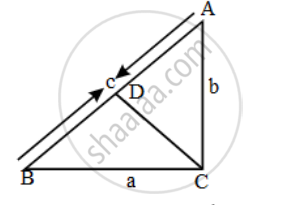Share

# ABC is a right triangle right-angled at C. Let BC = a, CA = b, AB = c and let p be the length of perpendicular from C on AB, prove that - CBSE Class 10 - Mathematics

#### Question

ABC is a right triangle right-angled at C. Let BC = a, CA = b, AB = c and let p be the length of perpendicular from C on AB, prove that

(i) cp = ab

(ii) 1/p^2=1/a^2+1/b^2

#### Solution

(i) Let CD ⊥ AB. Then, CD = pAB=c,BC=a,AC=b and CD=p

Area of triangle ABC=1/2ABxxCD=1/2xxcxxp=1/2cp

Also,

Area of triangle ABC=1/2BCxxAC=1/2xxaxxb=1/2ab

∴ \frac { 1 }{ 2 } cp = \frac { 1 }{ 2 } ab

⇒ cp = ab

(ii) Since ∆ABC is right triangle right-angled at C.

∴ AB^2 = BC^2 + AC^2

⇒ c^2 = a^2 + b^2

=>((ab)/p)^2=a^2+b^2

=>(a^2b^2)/p^2=a^2+b^2

=>1/p^2=(a^2+b^2)/(a^2b^2)=>1/p^2=1/b^2+1/a^2

=>1/p^2=1/a^2+1/b^2

Is there an error in this question or solution?

#### Video TutorialsVIEW ALL 

Solution ABC is a right triangle right-angled at C. Let BC = a, CA = b, AB = c and let p be the length of perpendicular from C on AB, prove that Concept: Pythagoras Theorem.
S